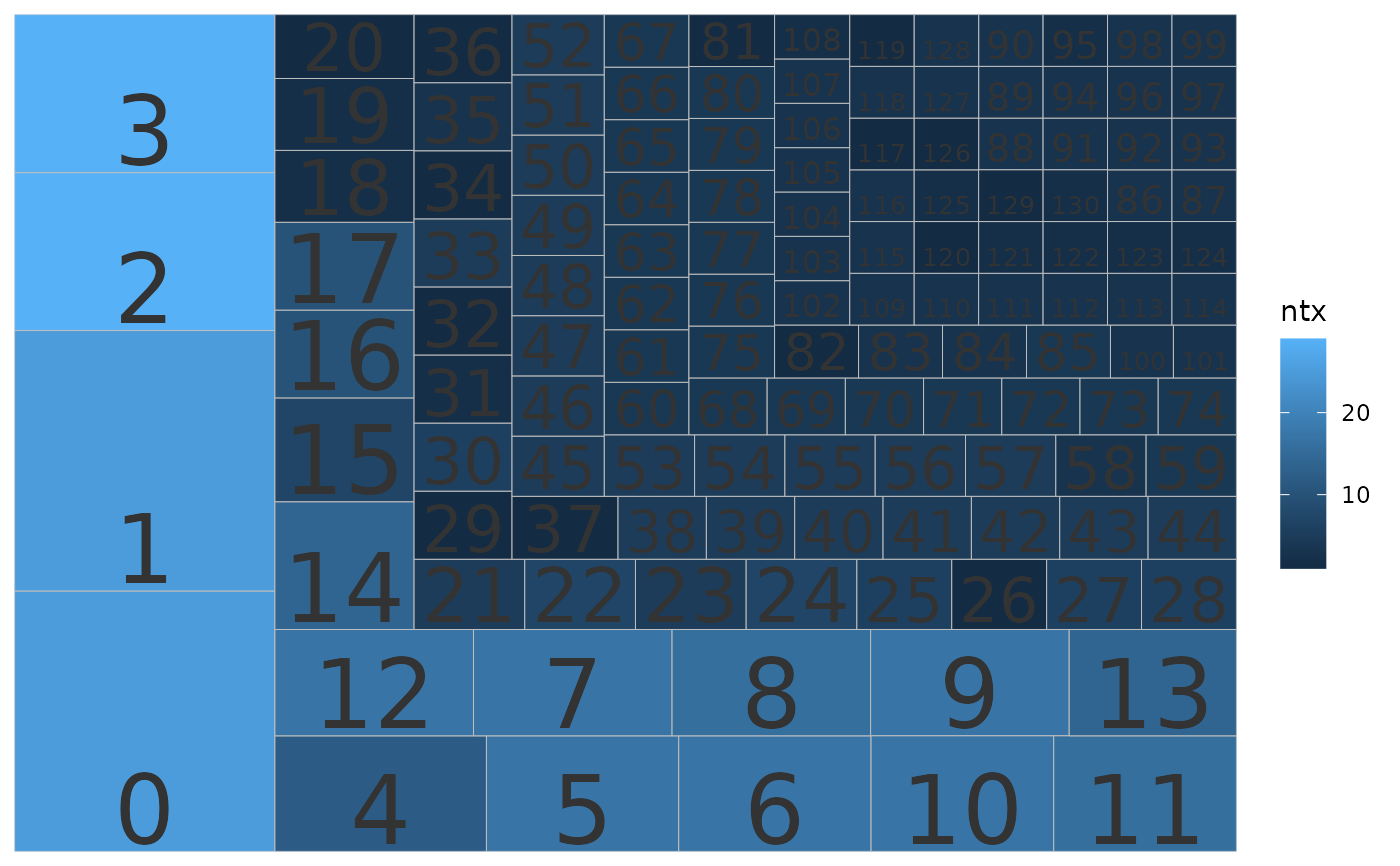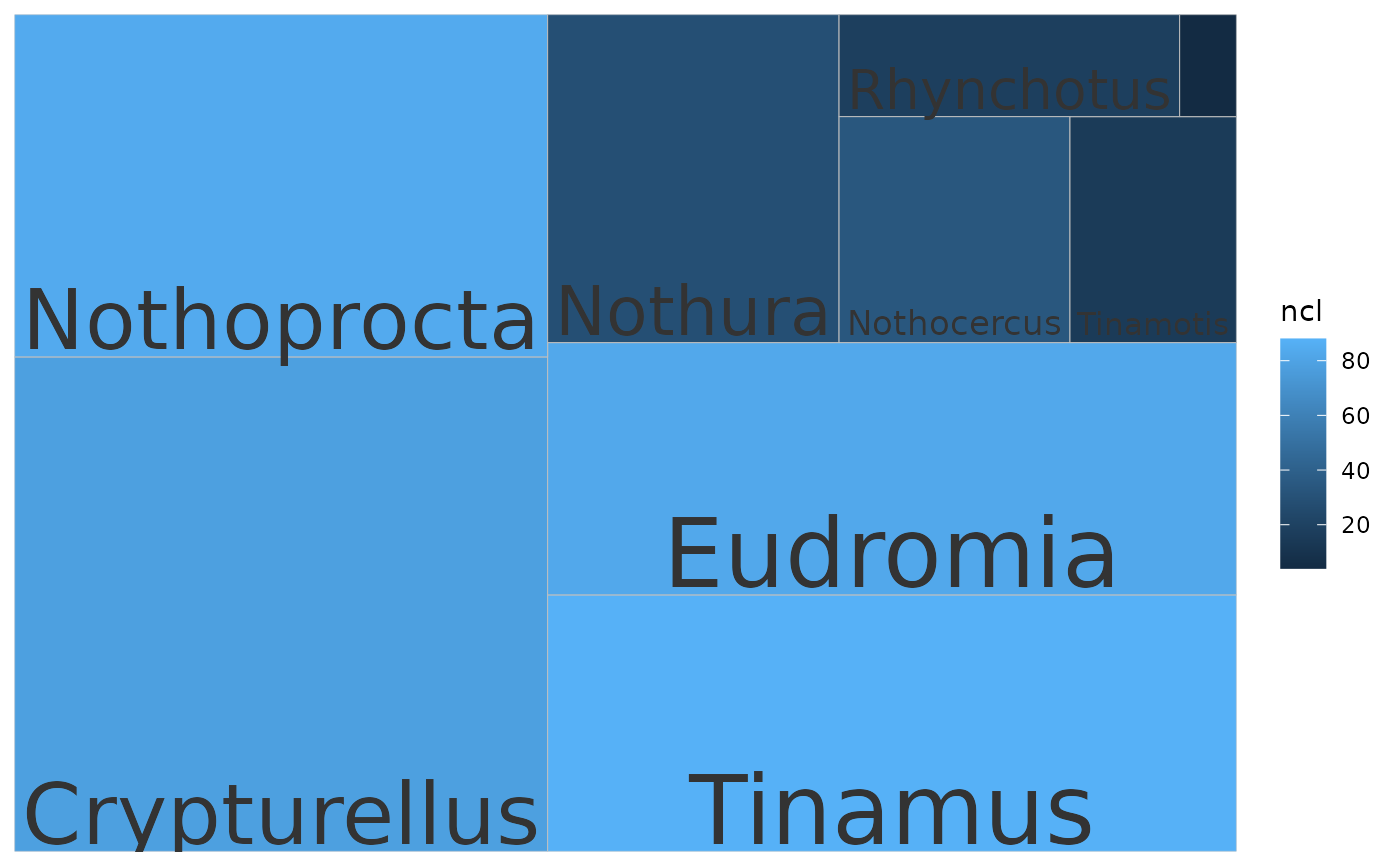Treemaps show relative size with boxes. The user can explore which taxa or clusters are most represented either by sequence or cluster number. If cluster IDs are provided, the plot is made for clusters. If taxonomic IDs are provided, the plot is made for taxa.

## Usage

``````plot_phylota_treemap(
phylota,
cids = NULL,
txids = NULL,
cnms = cids,
txnms = txids,
with_labels = TRUE,
area = c("ntx", "nsq", "ncl"),
fill = c("NULL", "typ", "ntx", "nsq", "ncl")
)``````

## Arguments

phylota

Phylota object

cids

Cluster IDs

txids

Taxonomic IDs

cnms

Cluster names

txnms

Taxonomic names

with_labels

Show names per box?

area

What determines the size per box?

fill

What determines the coloured fill per box?

geom_object

## Details

The function can take a long time to run for large Phylota objects over many taxonomic IDs because searches are made across lineages. The idea of the function is to assess the data dominance of specific clusters and taxa.

Other tools-public: `calc_mad()`, `calc_wrdfrq()`, `drop_by_rank()`, `drop_clstrs()`, `drop_sqs()`, `get_clstr_slot()`, `get_nsqs()`, `get_ntaxa()`, `get_sq_slot()`, `get_stage_times()`, `get_tx_slot()`, `get_txids()`, `is_txid_in_clstr()`, `is_txid_in_sq()`, `list_clstrrec_slots()`, `list_ncbi_ranks()`, `list_seqrec_slots()`, `list_taxrec_slots()`, `plot_phylota_pa()`, `read_phylota()`, `write_sqs()`

## Examples

``````data("tinamous")
# Plot clusters, size by n. sq, fill by n. tx
p <- plot_phylota_treemap(phylota = tinamous, cids = tinamous@cids,
area = 'nsq', fill = 'ntx')
print(p)# Plot taxa, size by n. sq, fill by ncl
txids <- get_txids(tinamous, txids = tinamous@txids, rnk = 'genus')
txids <- txids[txids !=  '']
txids <- unique(txids)
txnms <- get_tx_slot(tinamous, txids, slt_nm = 'scnm')
p <- plot_phylota_treemap(phylota = tinamous, txids = txids, txnms = txnms,
area = 'nsq', fill = 'ncl')
print(p)``````